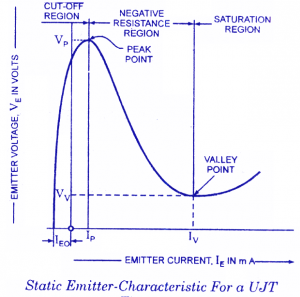# V-I characteristic of unijunction transistor (UJT)

The unijunction transistor characteristic is shown in below figure,The emitter voltage VE is applied between emitter E and base B1. VE is positive with respect B1. Let increasing this voltage from zero. The P-N junction is reverse biased and emitter current IE is negative as long as the emitter voltage VE < η VBB as shown by the curve PS. The current flowing are very close to the reverse leakage current of PN junction reverse biased. At point B, the emitter voltage VE is equal to ηVBB +VD the PN junction is forward biased and UJT starts conducting. The point B is known as the peak point and the voltage and current across its known as peak point voltage (VP) and peak point current (IP) respectively. The emitter voltage VE decreases with increase in emitter current IE. Due to drop in resistance rb1 with increase in the value of IE reduction in voltage across UJT. Thus the device exhibits a negative resistance region as shown by the curve BC. The entire base region is saturated at point C and rb1 does not decrease any more. As shown in curve CQ, the rise in voltage VE so further increase in IE. The point C is known as valley voltage and the voltage and current across is known as valley voltage (VV) and valley current (IV).